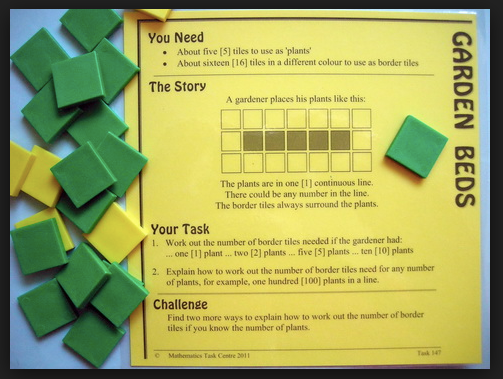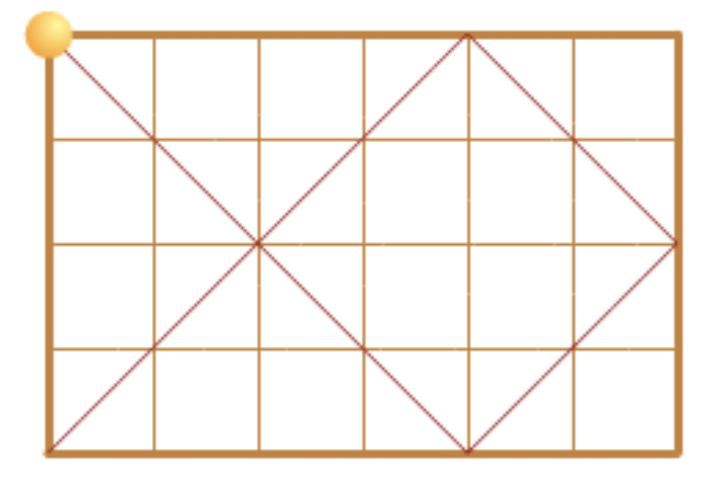# STEM Lesson Plan & Activities - SPHERO Year 7Sphero and Mathematics – Measurement – Yr 7

Lesson zero

Sphero familiarisation

This lesson enables students just to play and explore before the formal lesson starts

Lesson one

Structured task to test dexterity with tool

Linked to measurement outcomes with regular and irregular shapes

Basic coding

Area and Perimetre

https://edu.sphero.com/cwists/preview/6920x

Lesson Two

Revisit last lesson skills

Reinforce basic coding

Repeat but create shapes with perimeter of 30 units

How many different area?

Max and Min

Lesson 3

Repeat with fixed area and changing perimeter

Exploring a different concept with the same tool

Lesson 4/5/6

Let’s try the garden bed task

Use scale ratio 1:5 to enlarge the garden bed from below

Insert ratio lesson in mathematicsThis is extending the mathematics content to include scale and ratio as the tool needs a bigger area to allow for error

Lesson 7

Teach Billiard Problem on paper

https://mathforlove.com/lesson/billiard-ball-problem/Lesson 8/9

Now create and code this to work with the sphero

This will enable the students to test out the accuracy of their predictions and the accuracy of their coding

All should exit either TL TR BL BR

Is there a pattern